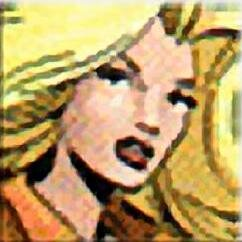Lady Aleena

# Expanded alignments

Are there times when you can't choose an alignment for your character with so few choices? That has come to an end. Here are 112 new alignments to choose from. A (+) next to an alignment means your character is true (alignment). A (-) next to an alignment means your character is just not that alignment. An alignment in parentheses means your character has those tendencies.

This chart has been copied and modified here.

## The Alignments

 L+G+ LG+ L(N)G+ -CG+ N(L)G+ NG+ N(C)G+ -LG+ C(N)G+ CG+ C+G+ L+G LG L(N)G -CG N(L)G NG N(C)G -LG C(N)G CG C+G L+G(N) LG(N) L(N)G(N) -CG(N) N(L)G(N) NG(N) N(C)G(N) -LG(N) C(N)G(N) CG(N) C+G(N) L+-E L-E L(N)-E -C-E N(L)-E N-E N(C)-E -L-E C(N)-E C-E C+-E L+N(G) LN(G) L(N)N(G) -CN(G) N(L)N(G) NN(G) N(C)N(G) -LN(G) C(N)N(G) CN(G) C+N(G) L+N LN L(N)N -CN N(L)N N+ N(C)N -LN C(N)N CN C+N L+N(E) LN(E) L(N)N(E) -CN(E) N(L)N(E) NN(E) N(C)N(E) -LN(E) C(N)N(E) CN(E) C+N(E) L+-G L-G L(N)-G -C-G N(L)-G N-G N(C)-G -L-G C(N)-G C-G C+-G L+E(N) LE(N) L(N)E(N) -CE(N) N(L)E(N) NE(N) N(C)E(N) -LE(N) C(N)E(N) CE(N) C+E(N) L+E LE L(N)E -CE N(L)E NE N(C)E -LE C(N)E CE C+E L+E+ LE+ L(N)E+ -CE+ N(L)E+ NE+ N(C)E+ -LE+ C(N)E+ CE+ C+E+